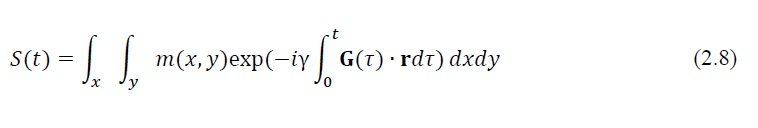## Spatial Encoding and k-Space Formalism

Following slice selection, the in-plane spatial information now can be further encoded with two additional gradients, known as frequency-encoding and phase-encoding gradients. In 2D imaging, the received MRI signal can be described asHere m(x, y) represents the spin density in the 2D object to be imaged. Considering only the x and y gradient fields Gx(t) and Gy(t), Eq. (2.8) becomesSettingEq. (2.9) can be rewritten asHere kx and ky are the spatial-frequency variables in a two-dimensional space that is known as k-space. Eq. (2.11) is usually referred as the signal equation, which suggests that the MRI signal represents the spatial Fourier transform of the object being imaged. The signal equation can be extended into three dimensions asHere additional phase-encoding is employed along the partition dimension

https://search.proquest.com/openview/eec314ed838aba2c6d50ce7f75b7e2c2/1.pdf?pq-origsite=gscholar&cbl=18750&diss=y